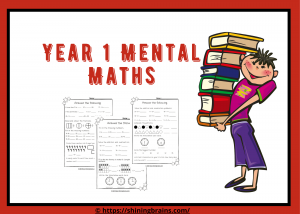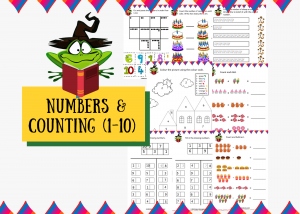# Single Digit Addition and Subtraction | Vertical One-Digit Equations

Single-digit addition and subtraction worksheets will be helpful for the beginning students. The worksheets are perfect for Kindergarten and year1 students. Let your kids practise addition and subtraction through these vertical equation worksheets. In addition to this, there are also mixed problems worksheets given in the end for practice.

Two-digit addition and Subtraction | vertical equations

Three-digit addition and subtraction with and without regrouping

Print the PDF file by clicking the pictures below.Mental maths makes the child proficient with numbers. It lays the keystone of more complex maths. Do you want to test your kid’s mental maths skills? Here are some free printable year1 maths worksheets. These worksheets are specially designed for year1 and year2 students. Moreover, these worksheets will access your kids understanding of maths concepts.

## Single Digit Subtraction WorksheetsUse these engaging printable worksheets to teach you kids counting. Make your child practise number recognition and counting with these kindergarten maths worksheets. These worksheets include number recognition, picture addition and counting activities. These Colourful maths PDF are a great way to train your kids with numbers.

## Addition and Subtraction Worksheets | Mixed Problems

Also, check: CVC Words Worksheets